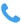+91 - 98400 38275

EMI CALCULATOR

Loan Amount*

Loan Tenure (Months)*

Interest Rate (Reducing)*

%

Monthly Payment (EMI)

See:
 Calculated Monthly EMI Total Amount with Interest Flat Interest Rate PA Flat Interest Rate PM Total Interest Amount Yearly Interest Amount